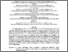# Optimal design of orders of DFrFTs for sparse representations

Zhang, X.-Z., Ling, B.W.-K., Tao, R., Yang, Z.-J., Woo, W.-L., and Teo, K.L., 2018. Optimal design of orders of DFrFTs for sparse representations. IET Signal Processing. ISSN 1751-9675Preview
Text
11028_1146a_Sanei.pdf - Post-print

## Abstract

This paper proposes to use a set of discrete fractional Fourier transform (DFrFT) matrices with different rotational angles to construct an overcomplete kernel for sparse representations of signals. The design of the rotational angles is formulated as an optimization problem as follows. The sum of the L1 norms of both the real part and the imaginary part of transformed vectors is minimized subject to different values of the optimal rotational angles. In order to avoid all the optimal rotational angles within a small neighbourhood, constraints on the sum of the L1 norms of both the real part and the imaginary part of the product of the individual optimal DFrFT matrices and training vectors being either stationary or nondifferentiable are imposed. Solving this optimization problem is very challenging not only because of the nonsmooth and the nonconvex nature of the problem, but also due to expressing the optimization problem in a nonstandard form. To solve the problem, first it is shown in this paper that this design problem is equivalent to an optimal sampling problem as follows. The absolute sum of the L1 norms of both the real part and the imaginary part of the frequency responses of a set of filters at the optimal sampling frequencies is minimized subject to similar constraints. Second, it is further shown that the optimal sampling frequencies are the roots of a set of harmonic functions. As the frequency responses of the filters are required to be computed only at frequencies in a discrete set, the globally optimal rotational angles can be found very efficiently and effectively.

Item Type: Journal article
Publication Title: IET Signal Processing
Creators: Zhang, X.-Z., Ling, B.W.-K., Tao, R., Yang, Z.-J., Woo, W.-L., Sanei, S. and Teo, K.L.
Publisher: The Institution of Engineering and Technology (IET)
Date: 27 April 2018
ISSN: 1751-9675
Identifiers:
NumberType
10.1049/iet-spr.2017.0283DOI
Divisions: Schools > School of Science and Technology
Record created by: Linda Sullivan
Date Added: 10 May 2018 11:40Edit View